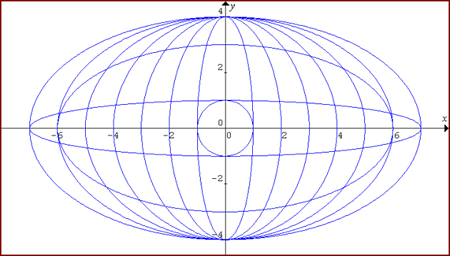#### You may also like### Cubic Spin

Prove that the graph of f(x) = x^3 - 6x^2 +9x +1 has rotational symmetry. Do graphs of all cubics have rotational symmetry?### Sine Problem

In this 'mesh' of sine graphs, one of the graphs is the graph of the sine function. Find the equations of the other graphs to reproduce the pattern.### Parabolic Patterns

The illustration shows the graphs of fifteen functions. Two of them have equations y=x^2 and y=-(x-4)^2. Find the equations of all the other graphs.

# Ellipses

##### Age 14 to 18Challenge Level

Here is a pattern for you to experiment with using graph drawing software.The equations of two of the graphs are: $$\frac{x^2}{36}+\frac{y^2}{16}=1$$ $$x^2 + y^2 = 1$$ Find the equations of the other 8 graphs in this pattern.

How do you know from their equations that all the graphs are symmetrical about both the y-axis and the x-axis?

Draw your own pattern of ellipses and circles.